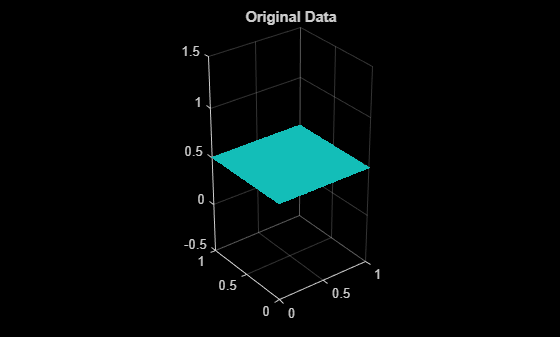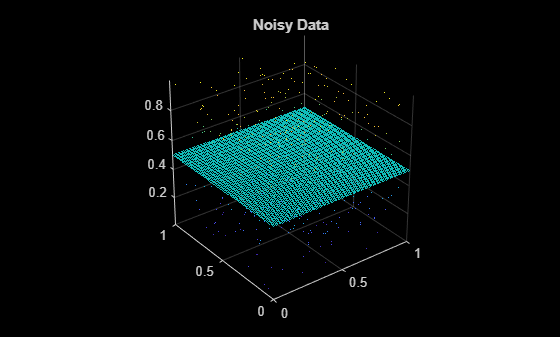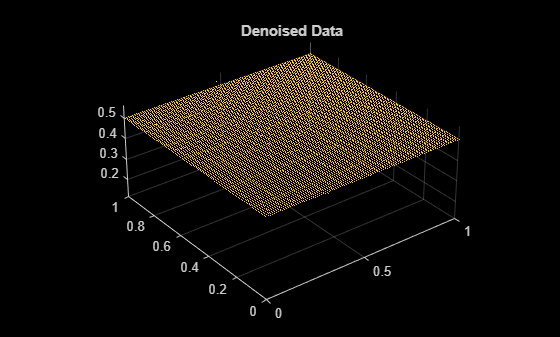pcdenoise

Remove noise from 3-D point cloud

Description

example

ptCloudOut = pcdenoise(ptCloudIn) returns a filtered point cloud that removes outliers.

[ptCloudOut,inlierIndices,outlierIndices] = pcdenoise(ptCloudIn) additionally returns the linear indices to the points that are identified as inliers and outliers.

[ptCloudOut,___] = pcdenoise(___Name,Value) uses additional options specified by one or more Name,Value pair arguments, using any of the preceding syntaxes.

Examples

collapse all

Create a plane point cloud.

gv = 0:0.01:1;
[X,Y] = meshgrid(gv,gv);
ptCloud = pointCloud([X(:),Y(:),0.5*ones(numel(X),1)]);

figure
pcshow(ptCloud);
title('Original Data');noise = rand(500, 3);
ptCloudA = pointCloud([ptCloud.Location; noise]);

figure
pcshow(ptCloudA);
title('Noisy Data');Remove outliers.

ptCloudB = pcdenoise(ptCloudA);

figure;
pcshow(ptCloudB);
title('Denoised Data');Input Arguments

collapse all

Point cloud, specified as a pointCloud object.

Name-Value Arguments

Specify optional comma-separated pairs of Name,Value arguments. Name is the argument name and Value is the corresponding value. Name must appear inside quotes. You can specify several name and value pair arguments in any order as Name1,Value1,...,NameN,ValueN.

Example: 'Threshold',1.0 sets the threshold to 1.0.

Number of nearest neighbor points, specified as the comma-separated pair consisting of 'NumNeighbors' and a positive integer in pixels. The value is used to estimate the mean of the average distance to neighbors of all points. Decreasing this value makes the filter more sensitive to noise. Increasing this value increases the number of computations.

Data Types: single | double

Outlier threshold, specified as the comma-separated pair consisting of 'Threshold' and a scalar. By default, the threshold is one standard deviation from the mean of the average distance to neighbors of all points. A point is considered to be an outlier if the average distance to its k-nearest neighbors is above the specified threshold.

Data Types: single | double

Output Arguments

collapse all

Filtered point cloud, returned as a pointCloud object.

Linear index of inlier points, returned as a 1-by-N vector.

Data Types: uint32

Linear index of outlier points, returned as a 1-by-N vector of linear indices.

Data Types: uint32

 Rusu, R. B., Z. C. Marton, N. Blodow, M. Dolha, and M. Beetz. “Towards 3D Point Cloud Based Object Maps for Household Environments”. Robotics and Autonomous Systems Journal. 2008.